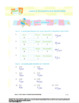# Grade 4:Decimals&Fractions:L4:Decimal Fraction Relationship Worksheet 4.NF.C.5&6Subject
Resource Type
File Type

Zip

(6 MB|8 pages)
Standards
Also included in:
1. Ready for the Bell math resources provide a comprehensive set of print, multimedia resources, and assessments with real world learning explorations.There are several types of activities in the Student Worksheets  that can be used for formative or summative assessment. They include:-Let’s Explore (Ex
\$18.00
\$16.20
Save \$1.80
2. Ready for the Bell math resources provide a comprehensive set of print, multimedia resources, and assessments with a real world learning explorations.There are several types of activities in the Student Worksheets  that can be used for formative or summative assessment. They include:-Let’s Explore (
\$93.00
\$83.70
Save \$9.30
• Product Description
• StandardsNEW

Ready for the Bell math resources provide a comprehensive set of print, multimedia resources, and assessments with real world learning explorations.

There are several types of activities in the Student Worksheets  that can be used for formative or summative assessment. They include:

-Let’s Explore (Exploration activities) – hands on active learning activities

-Let’s Practice – additional activities for students to practice

- Mixed Review – a series of questions that have been developed based on what students will have learned by this point as well as some questions that hit at areas that will be emerging in the future. You can use these Mixed Review questions as a formative assessment strategy to help you see the level of student understanding. You can also use some of these questions as an opportunity to extend the concept further with your students.

This Worksheet includes:

- Student Worksheet: 4 pages

- Answer Key for Worksheet: 4 pages

This Worksheet is part of the Math 4 lesson bundles. Click below to see these bundles.

Lesson 4 Packet: Decimal Fraction Relationships

Unit Packet: Decimals and Fractions

Common Core Alignment

CCSS.MATH.CONTENT.4.NF.C.5
Express a fraction with denominator 10 as an equivalent fraction with denominator 100, and use this technique to add two fractions with respective denominators 10 and 100.2 For example, express 3/10 as 30/100, and add 3/10 + 4/100 = 34/100.

CCSS.MATH.CONTENT.4.NF.C.6
Use decimal notation for fractions with denominators 10 or 100. For example, rewrite 0.62 as 62/100; describe a length as 0.62 meters; locate 0.62 on a number line diagram.

Use decimal notation for fractions with denominators 10 or 100. For example, rewrite 0.62 as 62/100; describe a length as 0.62 meters; locate 0.62 on a number line diagram.
Express a fraction with denominator 10 as an equivalent fraction with denominator 100, and use this technique to add two fractions with respective denominators 10 and 100. For example, express 3/10 as 30/100, and add 3/10 + 4/100 = 34/100.
Total Pages
8 pages
Included
Teaching Duration
45 minutes
Report this Resource to TpT
Reported resources will be reviewed by our team. Report this resource to let us know if this resource violates TpT’s content guidelines.